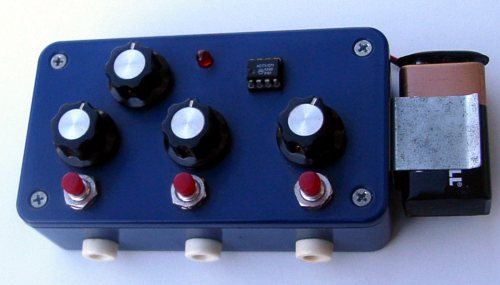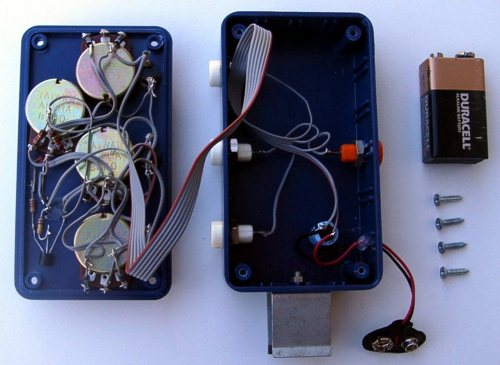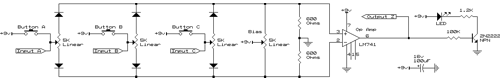# Artificial Neuron

Your brain is made up of neurons. Each neuron is a cell that sums its inputs, then if the total is greater than its threshold, it fires an output. That's basically all you and I are: 100 billion little adders, all running in parallel, cross-connected in interesting ways.

Since neurons are simple devices, they are easy to construct. I built one using an operational amplifier and a few variable resistors. It has three inputs which can either be controlled manually using push buttons or electrically using banana jacks. Each input is weighted positively or negatively using a potentiometer. The sum of the weighted inputs is compared against the threshold potentiometer. If the sum exceeds the threshold, a light turns on and power is sent to the output banana jack.If one were to build several of these boxes, one could use banana plugs to network them with each other. Build a few billion of them, connect them just right, tune all the dials, and one would have created a sentient creature. But even a single neuron is capable of an impressive range of functions. Here's a list of all configurations (with trivial permutations thereof removed) as computed by a Python script.

Formula Neuron Gate   Formula Neuron Gate
False 0   True 1
B   !B
A·B·C   !(A·B·C)
A+B+C   !(A+B+C)
A·B·!C   !(A·B·!C)
A+B+!C   !(A+B+!C)
A·!(B·C)   !(A·!(B·C))
A+!(B+C)   !(A+!(B+C))
A·(B+C)   !(A·(B+C))
A+(B·C)   !(A+(B·C))
A·(B+!C)   !(A·(B+!C))
A+(B·!C)   !(A+(B·!C))
(A·B)+(B·C)+(A·C)   !((A·B)+(B·C)+(A·C))
(A·B)+(B·!C)+(A·!C)   !((A·B)+(B·!C)+(A·!C))

Conspicuously absent from this table is exclusive or. A two-input XOR or XNOR requires two neurons [X=A·B, (A+B)·!X], although many sources claim three neurons [X=A·!B, Y=!A·B, X+Y].

## Sequential Logic

The table above lists a large number of combinational logic configurations; for each input there is one output. A neuron is also capable of sequential logic configurations; the output depends on the current inputs, and prior states. The banana jacks allow one to feed the output back into an input, thus forming a closed loop. The result is a single-bit memory circuit. A pulse to the first input will start a positive feedback loop, whereas a pulse to the second input will squelch the loop.

Negating the output (by negating all the weights and the threshold) results in a very fast oscillator.

## Software Simulator

Here's a software simulator of the neuron. Set the three input weights and the threshold, and the simulator will compute the output for all eight permutations of the inputs.

 Weight A: 3 2 1 0 -1 -2 -3 Weight B: 3 2 1 0 -1 -2 -3 Weight C: 3 2 1 0 -1 -2 -3 Threshold: 3.5 2.5 1.5 0.5 -0.5 -1.5 -2.5 -3.5
A B C Sum Output
0 0 0 ? ?
0 0 1 ? ?
0 1 0 ? ?
0 1 1 ? ?
1 0 0 ? ?
1 0 1 ? ?
1 1 0 ? ?
1 1 1 ? ?